# An alpha particle of charge +2e and mass 6.64 × 10-27 kg is moving in the...

An alpha particle of charge +2e and mass 6.64 × 10-27 kg is moving in the +x direction at 1.84×106 m/s. It enters a region where the electric field is 1779 N/C in the -x direction. How far will the alpha particle travel before it comes to a stop? Tries 0/5 How much time will elapse from when the alpha particle enters the electric field to when it stops?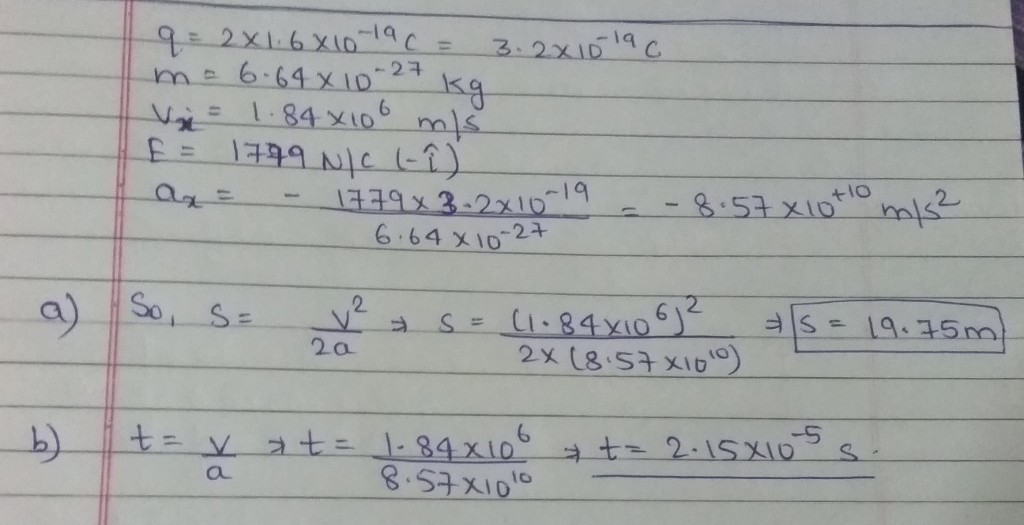#### Earn Coin

Coins can be redeemed for fabulous gifts.

Similar Homework Help Questions
• ### An alpha particle of charge +2e and mass 6.64 × 10-27 kg is moving in the...

An alpha particle of charge +2e and mass 6.64 × 10-27 kg is moving in the +x direction at 1.03×106 m/s. It enters a region where the electric field is 2835 N/C in the -x direction. How far will the alpha particle travel before it comes to a stop? How much time will elapse from when the alpha particle enters the electric field to when it stops?

• ### An alpha particle of charge +2e and mass 6.64 x 1027 kg is moving in the...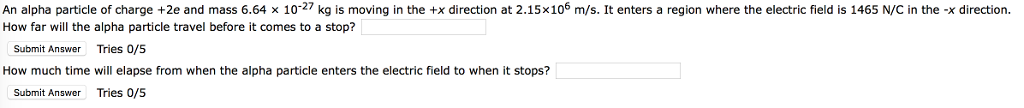An alpha particle of charge +2e and mass 6.64 x 1027 kg is moving in the +x direction at 2.15x106 m/s. It enters a region where the electric field is 1465 N/C in the -x direction. How far will the alpha particle travel before it comes to a stop? Submit Answer Tries 0/5 How much time will elapse from when the alpha particle enters the electric field to when it stops? Submit Answer Tries 0/5

• ### An alpha particle of charge +e and mass 6.64 x 127 kg is moving in the...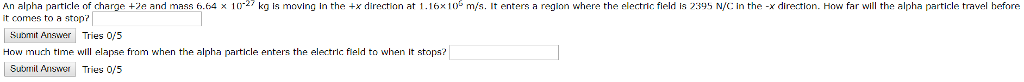An alpha particle of charge +e and mass 6.64 x 127 kg is moving in the +x direction at 1.16x10m/s. It enters a reginn where the electric field is 235 N/: in the -x direction. How far will the alpha particle travel hefore it comes to a stop? Submit Answer Tries 0/S How much time elapse fram when the alpha particle enters the electric field to when It stops? Sutbrmil Ariser Tries O/5

• ### An alpha-particle has a charge of +2e and a mass of 6.64 * 10^-27 kg. It...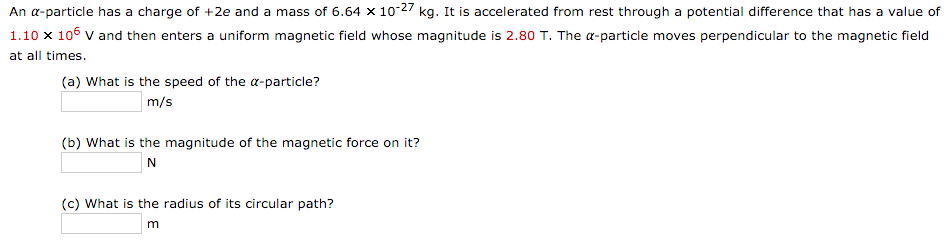An alpha-particle has a charge of +2e and a mass of 6.64 * 10^-27 kg. It is accelerated from rest through a potential difference that has a value of 1.10 * 10^6 V and then enters a uniform magnetic field whose magnitude is 2.80 T. The alpha-particle moves perpendicular to the magnetic field at all times. What is the speed of the alpha-particle What is the magnitude of the magnetic force on it What is the radius of its circular...

• ### An alpha-particle has a charge of +2e and a mass of 6.64 times 10^-27 kg. It...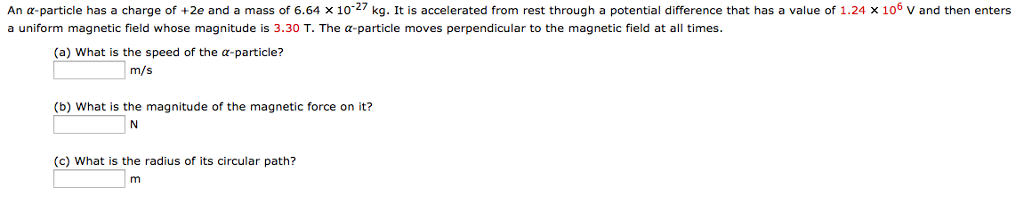An alpha-particle has a charge of +2e and a mass of 6.64 times 10^-27 kg. It is accelerated from rest through a potential difference that has a value of 1.24 times 10^6 v and then enters a uniform magnetic field whose magnitude is 3.30 T. The alpha-particle moves perpendicular to the magnetic field at all times. (a) What is the speed of the alpha-particle? m/s (b) What is the magnitude of the magnetic force on it? N (c) What is...

• ### An alpha particle (mass = 6.64⨯10-27 kg, charge = +2e) is accelerated by a potential drop...

An alpha particle (mass = 6.64⨯10-27 kg, charge = +2e) is accelerated by a potential drop V = -40 kV. What is its velocity upon emerging from the accelerating region?

• ### An α-particle has a charge of +2e and a mass of 6.64 × 10-27 kg. It...

An α-particle has a charge of +2e and a mass of 6.64 × 10-27 kg. It is accelerated from rest through a potential difference that has a value of 1.09 × 106 V and then enters a uniform magnetic field whose magnitude is 2.63 T. The α-particle moves perpendicular to the magnetic field at all times. What is (a) the speed of the α-particle, (b) the magnitude of the magnetic force on it, and (c) the radius of its circular...

• ### An α-particle has a charge of +2e and a mass of 6.64 × 10-27 kg. It...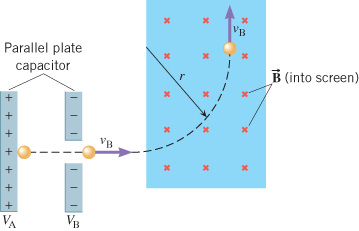An α-particle has a charge of +2e and a mass of 6.64 × 10-27 kg. It is accelerated from rest through a potential difference that has a value of 1.30 × 106 V and then enters a uniform magnetic field whose magnitude is 1.14 T. The α-particle moves perpendicular to the magnetic field at all times. What is (a) the speed of the α-particle, (b) the magnitude of the magnetic force on it, and (c) the radius of its circular...

• ### An α-particle has a charge of +2e and a mass of 6.64 10-27 kg. It is...

An α-particle has a charge of +2e and a mass of 6.64 10-27 kg. It is accelerated from rest through a potential difference that has a value of 1.08 106 V and then enters a uniform magnetic field whose magnitude is 2.30 T. The α-particle moves perpendicular to the magnetic field at all times. (a) What is the speed of the α-particle? m/s (b) What is the magnitude of the magnetic force on it? N (c) What is the radius...

• ### Alpha particles which have a charge +2e and mass 6.64 x 10-27 kg are initially at...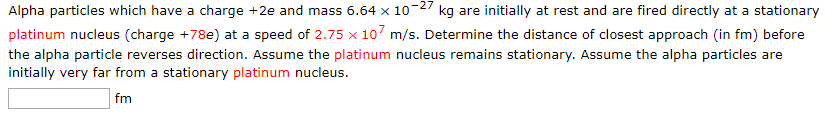Alpha particles which have a charge +2e and mass 6.64 x 10-27 kg are initially at rest and are fired directly at a stationary platinum nucleus (charge +78e) at a speed of 2.75 x 10 m/s. Determine the distance of closest approach (in fm) before the alpha particle reverses direction. Assume the platinum nucleus remains stationary. Assume the alpha particles are initially very far from a stationary platinum nucleus. fm